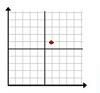# Plotting a point in quadrant 1 Online Quiz

#### Complete Python Prime Pack

9 Courses     2 eBooks

#### Artificial Intelligence & Machine Learning Prime Pack

6 Courses     1 eBooks

#### Java Prime Pack

9 Courses     2 eBooks

Following quiz provides Multiple Choice Questions (MCQs) related to Plotting a point in quadrant 1. You will have to read all the given answers and click over the correct answer. If you are not sure about the answer then you can check the answer using Show Answer button. You can use Next Quiz button to check new set of questions in the quiz.### Explanation

Step 1 − The x and y coordinates of the point are 1 and 3 respectively.

Step 2 − Plotting the point in the quadrant 1 we get the following.### Explanation

Step 1 − The x and y coordinates of the point are 2 and 8 respectively.

Step 2 − Plotting the point in the quadrant 1 we get the following.### Explanation

Step 1 − The x and y coordinates of the point are 3 and 10 respectively.

Step 2 − Plotting the point in the quadrant 1 we get the following.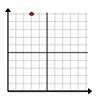### Explanation

Step 1 − The x and y coordinates of the point are 1 and 9 respectively.

Step 2 − Plotting the point in the quadrant 1 we get the following.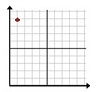### Explanation

Step 1 − The x and y coordinates of the point are 4 and 10 respectively.

Step 2 − Plotting the point in the quadrant 1 we get the following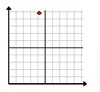### Explanation

Step 1 − The x and y coordinates of the point are 5 and 9 respectively.

Step 2 − Plotting the point in the quadrant 1 we get the following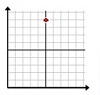### Explanation

Step 1 − The x and y coordinates of the point are 6 and 8 respectively.

Step 2 − Plotting the point in the quadrant 1 we get the following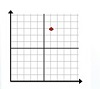### Explanation

Step 1 − The x and y coordinates of the point are 5 and 7 respectively

Step 2 − Plotting the point in the quadrant 1 we get the following### Explanation

Step 1 − The x and y coordinates of the point are 2 and 9 respectively.

Step 2 − Plotting the point in the quadrant 1 we get the following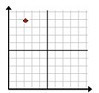### Explanation

Step 1 − The x and y coordinates of the point are 6 and 6 respectively.

Step 2 − Plotting the point in the quadrant 1 we get the following